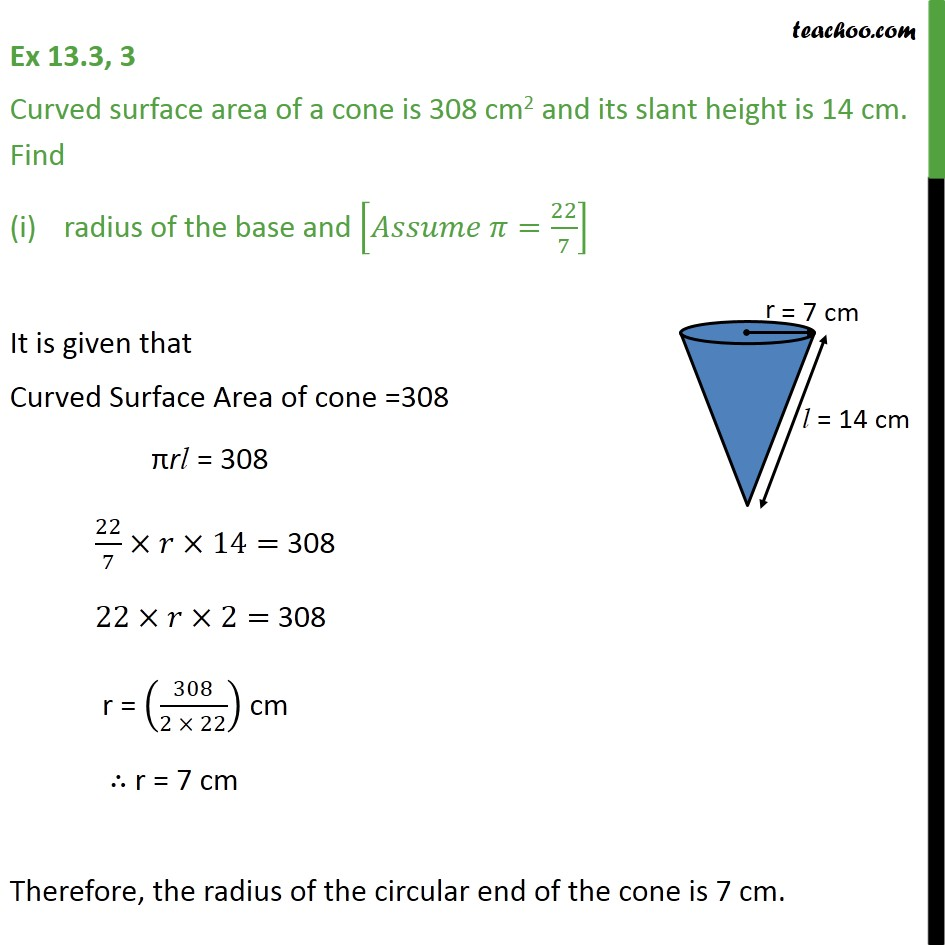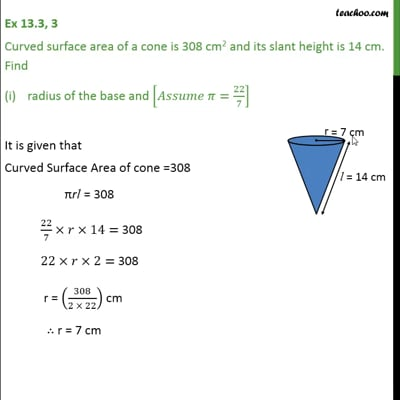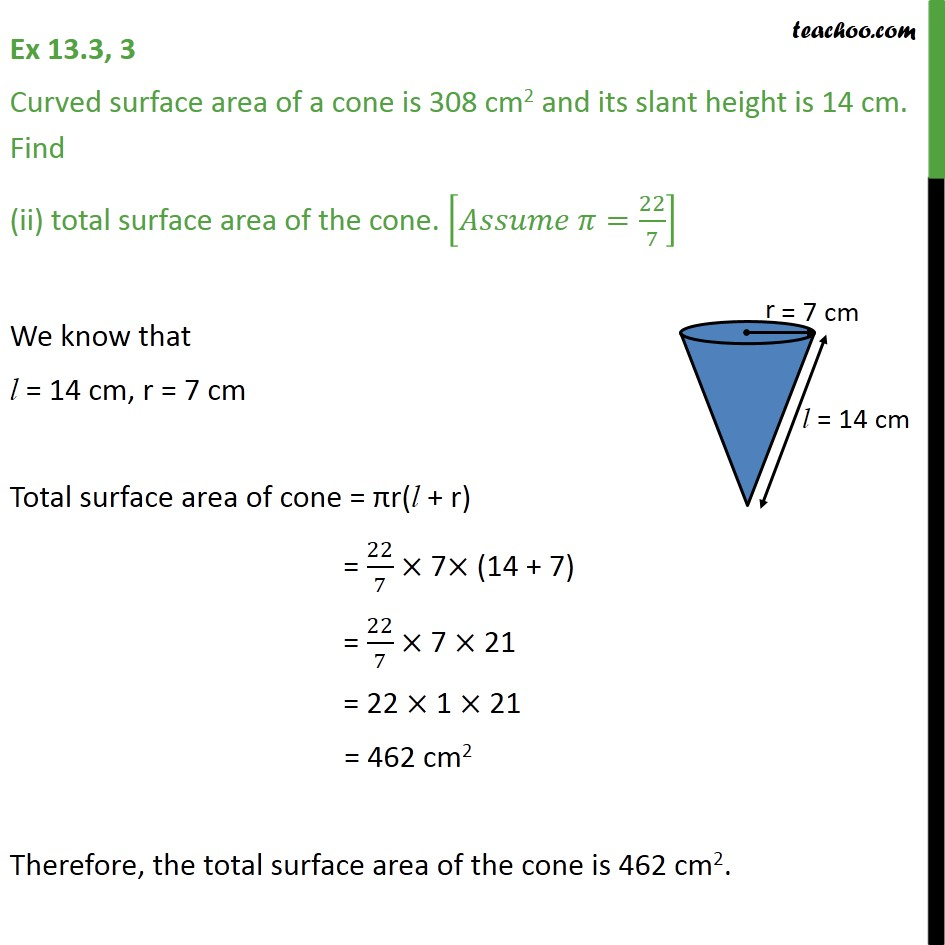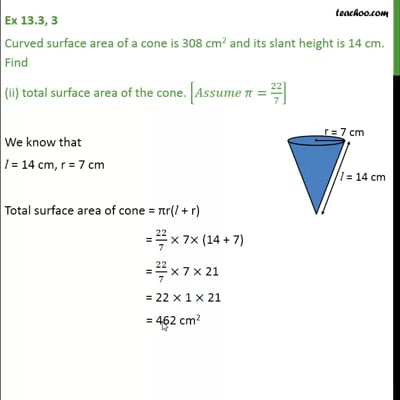Area Of Cone

Chapter 13 Class 9 Surface Areas and Volumes
Concept wiseThis video is only available for Teachoo black usersThis video is only available for Teachoo black users

Introducing your new favourite teacher - Teachoo Black, at only ₹83 per month

### Transcript

Ex 13.3, 3 Curved surface area of a cone is 308 cm2 and its slant height is 14 cm. Find radius of the base and [ =22/7] It is given that Curved Surface Area of cone =308 rl = 308 22/7 14= 308 22 2= 308 r = (308/(2 22)) cm r = 7 cm Therefore, the radius of the circular end of the cone is 7 cm. Ex 13.3, 3 Curved surface area of a cone is 308 cm2 and its slant height is 14 cm. Find (ii) total surface area of the cone. [ =22/7] We know that l = 14 cm, r = 7 cm Total surface area of cone = r(l + r) = 22/7 7 (14 + 7) = 22/7 7 21 = 22 1 21 = 462 cm2 Therefore, the total surface area of the cone is 462 cm2.